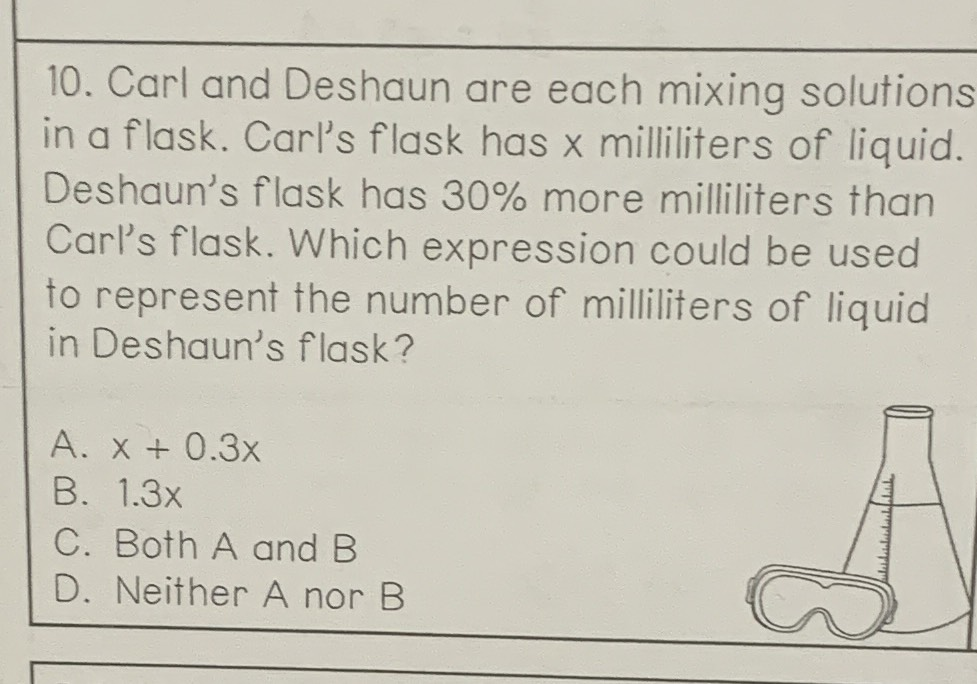### ¿Todavía tienes preguntas de matemáticas?

Pregunte a nuestros tutores expertos
Algebra
Pregunta10. Carl and Deshaun are each mixing solutions in a flask. Carl's flask has $$x$$ milliliters of liquid. Deshaun's flask has $$30 \%$$ more milliliters than Carl's flask. Which expression could be used to represent the number of milliliters of liquid in Deshaun's flask? A. $$x + 0.3 x$$

B. $$1.3 x$$ C. Both $$A$$ and B D. Neither A nor B